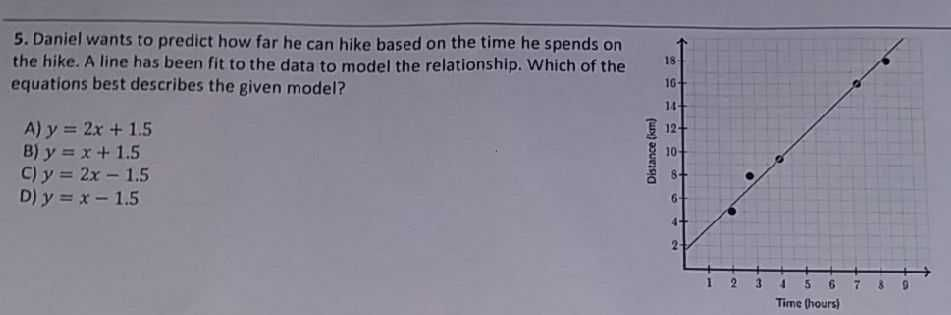### ¿Todavía tienes preguntas de matemáticas?

Pregunte a nuestros tutores expertos
Algebra
Pregunta5. Daniel wants to predict how far he can hike based on the time he spends on the hike. A line has been fit to the data to model the relationship. Which of the equations best describes the given model?

A) $$y = 2 x + 1.5$$

B) $$y = x + 1.5$$

C) $$y = 2 x - 1.5$$

D) $$y = x - 1.5$$